# Best Coursera Statistics Courses, Specialization and Training

Coursera is a well known and popular MOOC teaching platform that partners with top universities and organizations to offer online courses.

A typical course at Coursera includes pre recorded video lectures, multi-choice quizzes, auto-graded and peer reviewed assignments, community discussion forum and a sharable electronic course completion certificate.

You can study the course materials for free with the help of audit option.

But you have to pay if you want course certification and peer graded assignments.

Some Coursera courses facilitate onetime payment fee that lasts for 180 days.

If you enroll to courses that are part of a specialization, you have to opt for monthly subscription fee to have access to the courses.

Coursera hosts around a good number of courses, specializations, certificate programs and master’s degree in the field of probability and statistics.

All these courses are curated and taught by professors from world’s best universities.

Below are our best picks of Coursera probability and statistics courses if you come from a complete novice background in statistics or looking to learn statistical concepts for data analysis.

#### Basic Statistics – University of Amsterdam

The Coursera basic statistics course throws light on both the calculation and evaluation part of statistical concepts: descriptive statistics, basics of probability and inferential statistics.

It is the offered by the University of Amsterdam and is part of their methods and statistics in social media specialization.

Course Ratings: 4.7+ from 1,940+ students

#### Key Learning’s from the Course:

• Learn the basics of statistics
• Descriptive statistics methods: Cases, variables, mean, median, mode, standard deviation and variance
• Know probability basics such as calculating probabilities, probability and sampling distributions
• Introduction to inferential statistics methods
• Learn about confidence intervals and significance tests
• How to calculate and generate statistics results using statistical software

Who is this course best suited?  If you want to learn statistics using practical examples and case studies

Skills Gained from the course: Statistics, Confidence Interval, Statistical Hypothesis Testing and R Programming

Course Reviews: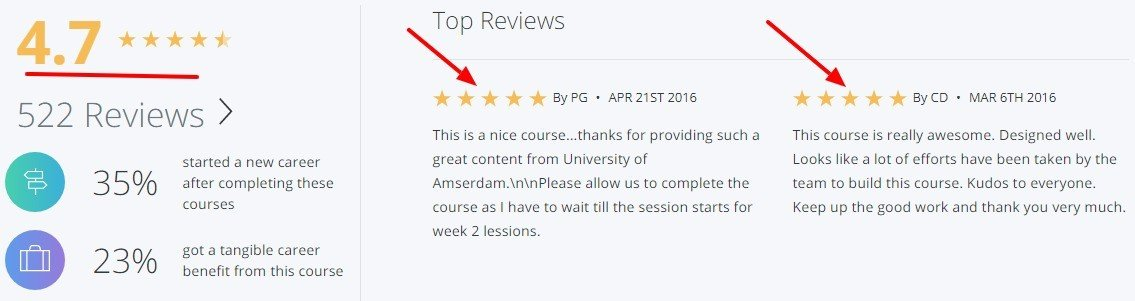#### Statistical Inference – Johns Hopkins University

The inferential statistics Coursera course offered by JHU gives a fundamental understanding on how to perform statistical inference from a set of data.

It is part of their popular data science specialization and will take you approx 16 hours to finish the course.

Course Ratings: 4.2+ from 3,039+ students

#### Key Learning’s from the Course:

• Describe variability, distributions, limits and confidence intervals
• Understand the process of drawing conclusions about populations or scientific truths about data
• Make informed data analysis decisions
• Use p-values, confidence intervals and permutation tests

Who is this course best suited?  Anyone interested in the technical understanding of statistical inference.

Skills Gained from the course: Statistics, Statistical Inference and Statistical Hypothesis Testing

Course Reviews: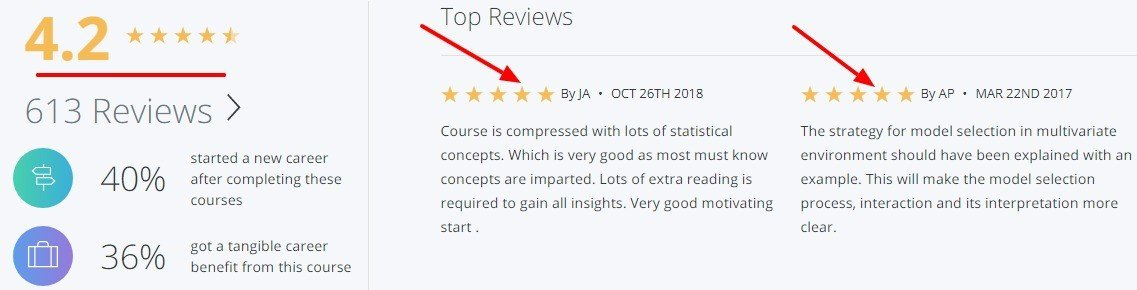#### Inferential Statistics – Duke University

Offered by Duke University, this is an equally good course to learn inferential statistics concepts at Coursera.

This beginner level course is part of the Coursera Statistics with R specialization and takes approx 25 hours to study the entire course.

Course Ratings: 4.8+ from 1,239+ students

#### Key Learning’s from the Course:

• Introduction of practical tools to perform data analysis
• Explore fundamentals statistical inference methods for numerical and categorical data
• How to set up and perform hypothesis tests, interpret p-values and report analysis
• Learn how to report estimate quantities that shows the uncertainty of the quantity of interest
• How to install and use R and RStudio and work on a data analysis project

Who is this course best suited?  Anyone who want to get in depth knowledge of statistics, inference and hypothesis.

Skills Gained from the course: Statistical Inference, Statistical Hypothesis Testing and R Programming

Course Reviews: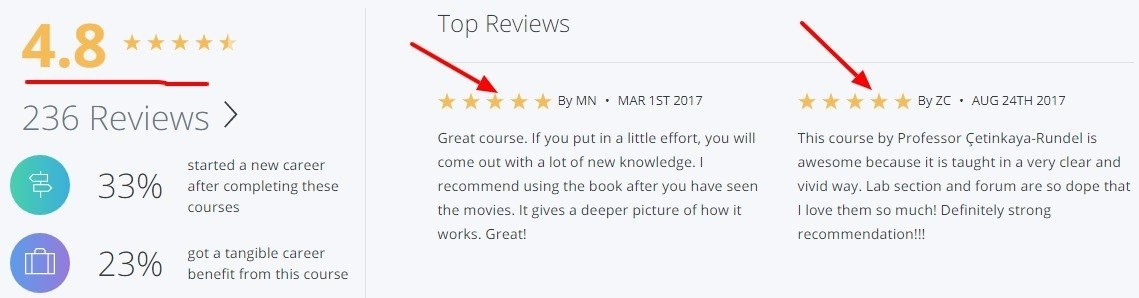#### Introduction to Probability and Data – Duke University

The Coursera Statistics with R course introduces exploratory data analysis techniques such as summary statistics and basic data visualization.

This is course 4 of the 5 series of Coursera Statistics with R specialization and takes around 22 hours to complete the course.

Course Ratings: 4.7+ from 2,755+ students

#### Key Learning’s from the Course:

• Introduction to probability and data
• Explore data through numerical summaries and visualizations
• Introduction to numerical and categorical data and inference
• How to install and use R and RStudio for assignments
• Introduction to probability, conditional probability, Bayes’ theorem and Bayesian inference
• How to use probability distributions: normal and binomial distributions

Who is this course best suited?  Highly recommended if you want to learn descriptive statistics and probability foundation with R programming language

Skills Gained from the course: Statistics, R Programming, RStudio and Exploratory Data Analysis

Course Reviews: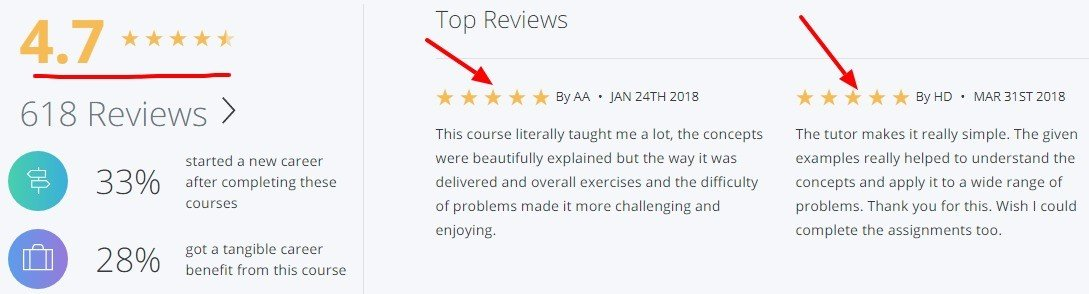#### Linear Regression for Business Statistics – RICE University

The linear regression for business statistics Coursera course talks about an important business statistics tool called Regression Analysis.

Part of the Business Statistics and Analysis Specialization offered by RICE University, it will take an approx 13 hours to complete the course.

Course Ratings: 4.8+ from 436+ students

#### Key Learning’s from the Course:

• Introduction to regression analysis
• Making inferences using the estimated model
• How to perform Hypothesis testing in Linear Regression
• Introduction to R-square and dummy variables regression
• Interpretation of coefficients and p-values in the presence of Dummy variables
• Know the various extensions used in regression analysis

Who is this course best suited?  If you want to gain a deep and solid understanding about linear regression

Skills Gained from the course: Log-Log Plot, Interaction (Statistics), Linear Regression and Regression Analysis

Course Reviews: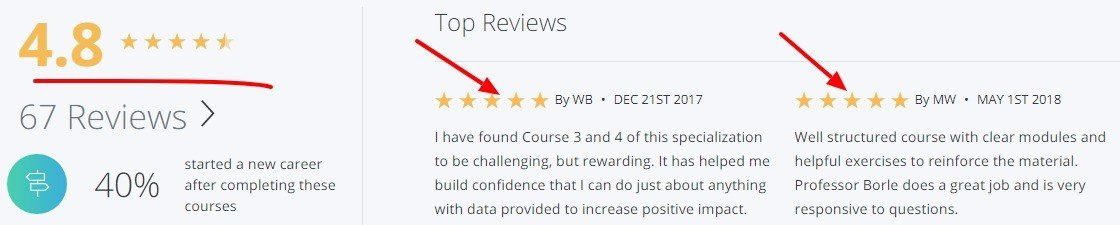#### Bayesian Statistics: From Concept to Data Analysis – University of California

The Coursera probability and statistics course helps you understand concepts of Bayesian approach, the key differences between Bayesian and Frequentist approaches and how to do data analysis using them.

Course Ratings: 4.6+ from 1,406+ students

#### Key Learning’s from the Course:

• Basics of Probability and Bayes’ Theorem
• Introduction to statistical inference concepts
• Learn methods for selecting prior distributions and building models for discrete data
• How to use conjugate model and Bayesian analysis for continuous data

Who is this course best suited? If you want to learn and practice Bayesian Inference in statistics

Skills Gained from the course: Statistics, Bayesian Statistics, Bayesian Inference and R Programming

Course Reviews:#### Bayesian Statistics – Duke University

The Coursera Bayesian statistics offered by Duke University is another alternative course to learn Bayesian analyses in depth.

This is the fourth course of the 5 course series of Coursera Statistics with R specialization and will take an approx 30 hours to complete it.

Course Ratings: 3.9+ from 505+ students

#### Key Learning’s from the Course:

• The basics of Bayesian Statistics concepts
• Understand and define the Bayesian Inference concepts
• Able to make optimal using Bayesian decision making, hypothesis testing and Bayesian testing
• Compare multiple hypothesis using Bayes factors
• Implement Bayesian model averaging, interpret Bayesian multiple linear regression and its relationship to the Frequentist linear regression approach
• Understand from other statistician on how they apply Bayesian statistics on their job

Who is this course best suited?  If you want to learn Bayesian statistics in detail

Skills Gained from the course: Bayesian Statistics, Bayesian Linear Regression, Bayesian Inference and R Programming

Course Reviews: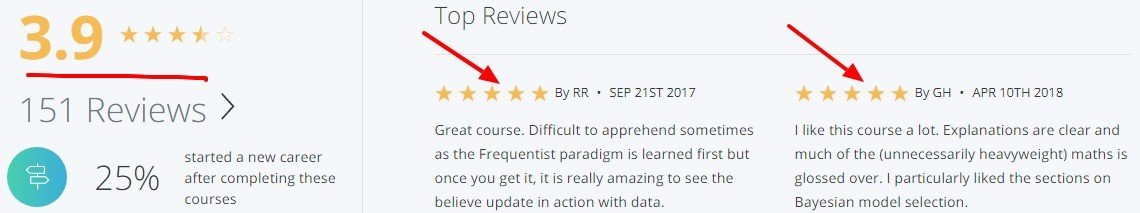#### Understanding and Visualizing Data with Python

The Coursera Statistics with Python course teaches learners how to apply statistical concepts such as data visualizations and data management using Python programming instead of R.

This is the introductory course of the Coursera Statistics with Python Specialization and takes an approx 19 hours to complete the entire course.

Course Ratings: 4.4+ from 47+ students

#### Key Learning’s from the Course:

• Properly identify various data types and understand the different uses for each
• Communicate statistical ideas clearly and concisely to a broad audience
• Create data visualizations and numerical summaries with Python
• Identify appropriate analytic techniques for probability and non-probability samples

Who is this course best suited?  If you want to learn statistics basics with Python programming

Skills Gained from the course: Statistics, Data Analysis, Python Programming and Data Visualization

Course Reviews: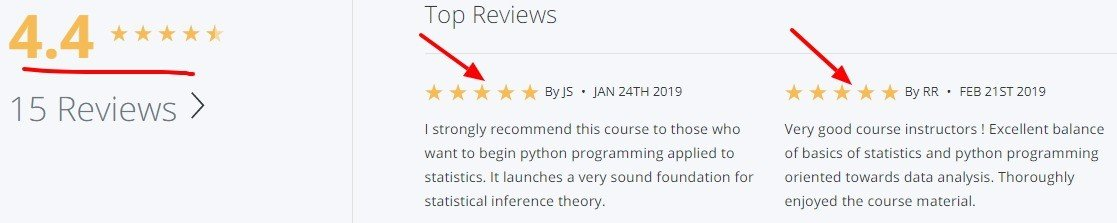Do you recommend any other Coursera statistics courses worth enrolling into? Let us know in the comments.

Happy Learning!